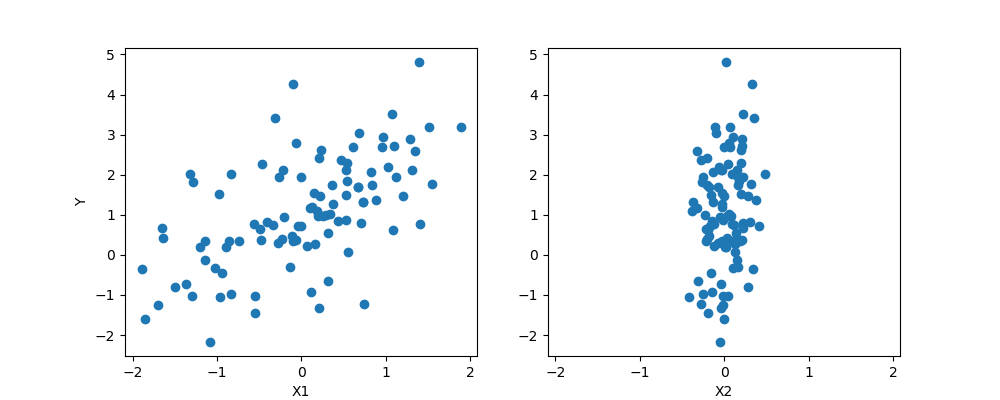# The Bayesian Thinking - III

Friday, June 22, 2018

Read the first part The Bayesian Thinking - I and the second part The Bayesian Thinking - II.

There’s no theorem like Bayes’ theorem
Like no theorem we know
Everything about it is a wow
Let out all that a priori feeling
You’ve been concealing right up to now!
George Box

In this post, we’ll do probabilistic programming in PyMC31, a python library for programming Bayesian analysis. We’ll discuss the Bayesian view of linear regression.

## Frequentist Linear Regression

In Frequentist Linear Regression,

$\hat{Y} = \hat{β_{0}} + \hatβ_{1}X_{1} + \hatβ_{2}X_{2} + ... + \hatβ_{p}X_{p}$ $Y = \hat{Y} + \epsilon = β^T X + \epsilon$

we use least squares fitting procedure to estimate regression coefficients $$\beta_{0}, \beta_{1}, \beta_{2}, ..., \beta_{p}$$ while minimizing the loss function using residual sum of squares:

$RSS = \sum_{i=1}^n(y_{i} - \hat{y_i})^2 = \sum_{i=1}^n(y_{i} - β^T x_{i})^2$

We calculate the maximum liklihood estimate of β, the value that is the most probable for X and y.

$\hat{β} = (X^T X)^{-1} X^T y$

This method of fitting the model parameters is called Ordinary Least Squares (OLS). We obtain a single estimate for the model parameters based on the training data.

## Bayesian Linear Regression

In the Bayesian view, the response, y, is not estimated as a single value, but is drawn from a probability distribution.

$y \sim N(\mu, \sigma^2)$ $\mu = \hat{α} + \hatβ_{1}X_{1} + \hatβ_{2}X_{2} + ... + \hatβ_{p}X_{p}$

where $$\sigma$$ represents the observation error.

The response, y is generated from a normal (Gaussian) distribution. In Bayesian linear regression, we determine the posterior distribution of model parameters rather than finding a single best value as in frequentist approach. Using the Bayes theorem, the posterior probability of parameters is given by

$P(β|y,X) = \frac{liklihood * prior}{normalization} = \frac{P(y|β,X)*P(β|X)}{P(y|X)}$

We include the guess, of what the parameters’ value can be, in our model, unlike the frequentist approach where everything comes from the data. If we don’t have any estimates, we can non-informative priors such as normal distribution.## Conclusion

The Bayesian reasoning is similar to our natural intuition. We start with an initial estimate, our prior, and as we gather more (data) evidence, we update our beliefs (prior), we update our model.

Footnotes:
1: PyMC3

References: